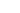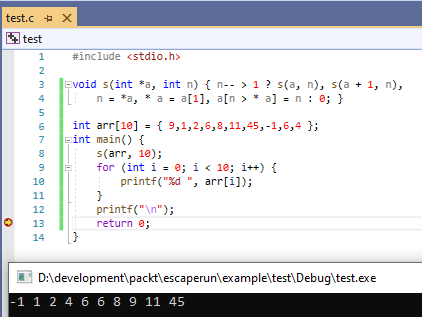### Tag: sort## Quicksort in C

Normally I wouldn’t mention a sorting algorithm, but by a lucky coincidence, when I had my interview before going to university (Queen’s University Belfast), the bloke who interviewed me back in 1977 was the inventor of Quicksort. Tony Hoare. He left very shortly after so I never got him as a lecturer,

As algorithms go, Quicksort is one of the faster. it works by splitting the list in two and then recursively sorting the two halves. The simplest sorting method Bubble sort uses a double loop and for very small numbers of items to sort is generally more efficient than Quicksort, but it doesn’t take long for Quicksort to catch up.

I was reminded of Quicksort by seeing this article and thought it would be interesting to do a series of test of sorting 5-50 items a few thousand times each in Quicksort and Bubblesort and see where the crossover point is. How many items is the minimum for Quicksort to become faster than Bubble sort?

If you take the Swap functions and BubbleSort from here and partition and quickSort functions from here and use my high precision timing code and add the code below then you can run the comparison. I did it twice, once in Debug and once in Release and the difference is much larger in release. I’ve zipped up the source code including the Visual C++ project code (It is C source code not C++ btw) and high performance timing code and put it on GitHub.

``````#define NUMLOOPS 5000

int main()
{
int startarr[]= { 86,91,50,5,79,64,2,57,26,63,12,61,12,92,40,38,11,
94,90,38,24,25,54,75,67,56,7,9,32,26,54,48,51,28,
90,50,37,53,8,75,30,25,59,57,92,42,25,33,84,46 };
int arr;
stopWatch stw;
double bTime, qTime;

bTime = 0.0;
qTime = 0.0;
for (int i = 3; i < 50; i++) {
printf("Bubble sort for %d = ", i);
for (int j = 1; j < NUMLOOPS; j++) {
memset(arr, 0, sizeof(arr));                     // Clear arr
memcpy(arr, startarr, sizeof(int) * i); // copy in i elements
startTimer(&stw);
bubbleSort(arr, i);
stopTimer(&stw);
bTime += getElapsedTime(&stw);
}
bTime /= NUMLOOPS;
printf("%12.10f.  ", bTime);
printf("Quicksort sort for %d = ", i);
for (int j = 1; j < NUMLOOPS; j++) {
memcpy(arr, startarr, sizeof(int) * i); // copy in i elements
startTimer(&stw);
quickSort(arr, 0, i - 1);
stopTimer(&stw);
qTime += getElapsedTime(&stw);
}
qTime /= NUMLOOPS;
printf("%12.10f\n", qTime);
}
return 0;
}``````

These are the first 15 and last 5 output lines in Release. The Bubble sort time for 7 is a bit of an oddity just for the actual numbers sorted. But its clear that by 11 values that Bubble sort is slower than QuickSort and the difference just gets worse up to 50. So up to 10 elements, Bubble Sort wins.

``````Bubble sort for 3 = 0.0000000358.  Quicksort sort for 3 = 0.0000000412
Bubble sort for 4 = 0.0000000407.  Quicksort sort for 4 = 0.0000000445
Bubble sort for 5 = 0.0000000474.  Quicksort sort for 5 = 0.0000000485
Bubble sort for 6 = 0.0000000677.  Quicksort sort for 6 = 0.0000000448
Bubble sort for 7 = 0.0000000191.  Quicksort sort for 7 = 0.0000000097
Bubble sort for 8 = 0.0000000405.  Quicksort sort for 8 = 0.0000000601
Bubble sort for 9 = 0.0000000510.  Quicksort sort for 9 = 0.0000000434
Bubble sort for 10 = 0.0000000568.  Quicksort sort for 10 = 0.0000000653
Bubble sort for 11 = 0.0000000633.  Quicksort sort for 11 = 0.0000000566
Bubble sort for 12 = 0.0000000702.  Quicksort sort for 12 = 0.0000000606
Bubble sort for 13 = 0.0000000845.  Quicksort sort for 13 = 0.0000000743
Bubble sort for 14 = 0.0000000931.  Quicksort sort for 14 = 0.0000001145
Bubble sort for 15 = 0.0000001040.  Quicksort sort for 15 = 0.0000000827
Bubble sort for 16 = 0.0000001142.  Quicksort sort for 16 = 0.0000000892
Bubble sort for 17 = 0.0000001448.  Quicksort sort for 17 = 0.0000000974
...
Bubble sort for 45 = 0.0000008478.  Quicksort sort for 45 = 0.0000002737
Bubble sort for 46 = 0.0000008561.  Quicksort sort for 46 = 0.0000002524
Bubble sort for 47 = 0.0000008948.  Quicksort sort for 47 = 0.0000002643
Bubble sort for 48 = 0.0000009421.  Quicksort sort for 48 = 0.0000003147
Bubble sort for 49 = 0.0000010042.  Quicksort sort for 49 = 0.0000002804``````## A very small sort in CI came across this article on efforts to make the smallest sort function in C.  Being cynical I did wonder if it would compile and run. I mean 56 bytes is kind of short!

To get it to compile in VS 2019 I had to add a couple of changes, mainly ints and the void. This is it wrapped in a program. The screenshot shows it running and the output at the bottom. Very clever!

The sort function is that void s(… at the top.

``````#include <stdio.h>

void s(int *a, int n) { n-- > 1 ? s(a, n), s(a + 1, n),
n = *a, * a = a, a[n > * a] = n : 0; }

int arr = { 9,1,2,6,8,11,45,-1,6,4 };
int main() {
s(arr, 10);
for (int i = 0; i < 10; i++) {
printf("%d ", arr[i]);
}
printf("\n");
return 0;
}``````

What I didn’t show you is the number of times Windows defender went ape and declared it a trojan, every time it compiled! My PC is kept pretty bug free and this is a false positive…